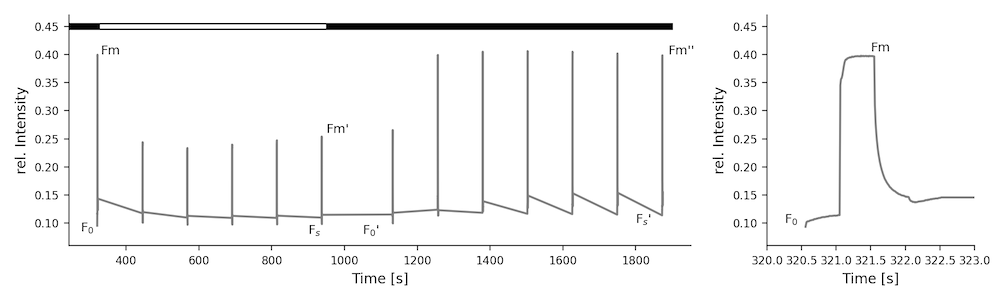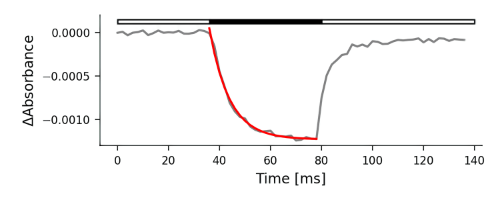# # References & Parameters

Note

This collection of references and equations is in no way complete nor is it intended to be. It is just a guideline to get you started in case you are working on your own calculations. If you use the equations, make sure to cite the publications accordingly.

## # Reviews & Articles

For a general purpose overview of fluorescence- and absorbance-based photosynthetic parameters, and their interpretation, we recommend the following sources:

Kramer, D.M., Cruz, J.A. and Kanazawa, A. (2003) Balancing the central roles of the thylakoid proton gradient. Trends Plant Sci. 8: 27-32 doi:10.1016/S1360-1385(02)00010-9 (opens new window)

Baker, N.R., Harbinson, J., and Kramer, D.M. (2007) Determining the limitations and regulation of photosynthetic energy transduction in leaves. Plant Cell Environ. 30: 1107-1125 doi:10.1111/j.1365-3040.2007.01680.x (opens new window)

Baker, N.R. (2008) Chlorophyll fluorescence: A probe of photosynthesis in vivo. Annu. Rev. Plant Biol. 59: 89-113 doi:10.1146/annurev.arplant.59.032607.092759 (opens new window)

Kramer, D. M., and Evans, J. R. (2011). The importance of energy balance in improving photosynthetic productivity. Plant Physiol. 155, 70–8. doi:10.1104/pp.110.166652 (opens new window).

Kalaji, H. M., Schansker, G., Ladle, R. J., Goltsev, V., Bosa, K., Allakhverdiev, S. I., et al. (2014) Frequently asked questions about in vivo chlorophyll fluorescence: practical issues. Photosynthesis Research 122, 121–158.doi:10.1007/s11120-014-0024-6 (opens new window).

Cruz, J. A., Savage, L. J., Zegarac, R., Hall, C. C., Satoh-Cruz, M., Davis, G. A., et al. (2016). Dynamic Environmental Photosynthetic Imaging Reveals Emergent Phenotypes. Cell Syst. 2, 365–377. doi:10.1016/j.cels.2016.06.001 (opens new window).

Kalaji, H. M., Schansker, G., Brestic, M., Bussotti, F., Calatayud, A., Ferroni, L., et al. (2016) Frequently asked questions about chlorophyll fluorescence, the sequel. Photosynthesis Research 132, 13–66. doi:10.1007/s11120-016-0318-y (opens new window).

Cruz, J. A. & Avenson, T. J. (2021) Photosynthesis: a multiscopic view. J Plant Res 134, 665–682. doi:10.1007/s10265-021-01321-4 (opens new window).

## # Environmental Parameters

### # Light

Parameter Details
Light Intensity (PAR) Photosynthetically active radiation. Fraction of the incoming light (400 - 700 nm) which can be utilized for photosynthesis; µmol photons × s⁻¹ × m⁻²
R The raw amount of red light captured by the PAR sensor
G The raw amount of green light captured by the PAR sensor
B The raw amount of blue light captured by the PAR sensor

### # Atmospheric Parameters

Parameter Details
Ambient Humidity Relative humidity in percent (%)
Ambient Pressure Atmospheric pressure (mbar) - This value is not corrected to sea level as found in weather reports
Ambient Temperature Ambient temperature in degree Celsius (℃)

### # Leaf Parameters

Parameter Details
contactless_temp Surface temperature in degree Celsius (℃)
Leaf Temperature Differential Leaf Temperature ( or contactless_temp) minus Ambient Temperature, negative numbers mean that the leaf is cooler than the surrounding air and vice versa.
Thickness The thickness of the leaf as measured by the Hall Effect sensor in (µm)

### # Positional Information

Parameter Details
angle_direction, compass_direction Abbreviated cardinal direction (e.g. NW - North West)
compass Cardinal direction in degrees from North
Leaf Angle The angle of the leaf, from 0 - 90 degrees
roll Roll is the angle the Instrument is held along the long axis
pitch Pitch is the angle the Instrument is held along the short axis

## # Chlorophyll Fluorescence (PAM)

Here you find commonly used equations to derive photosynthetic parameters from fluorescence changes measured using the Pulse-Amplitude-Modulation (PAM) method. Please be aware, that depending on the literature, the same parameters can have multiple names.Fluorescence quenching analysis using modulated fluorescence. Actinic light intensity 250 µmol photons × s⁻¹ × m⁻².

### # Yield and Electron Transfer

#### # Maximum Quantum Efficiency

(1) $\text{F}_{v} / \text{F}_{m} = \frac{ F_{m} - F_{0}}{Fm}$

#### # Quantum Yield

(2) $\Phi_{II} = \frac{F_{m}' - F_{s}}{F_{m}'}$

• Genty, B., Briantais, J.-M. & Baker, N.R. (1989). The relationship between the quantum yield of photosynthetic electron transport and quenching of chlorophyll fluorescence Biochimica et Biophysica Acta (BBA) - General Subjects 990(1), pp.87–92. doi:10.1016/S0304-4165(89)80016-9 (opens new window).

#### # Linear Electron Flow

(3) $\text{LEF} = \Phi_{II} \times \text{PAR} \times 0.4$

#### # Non-regulatory Energy Dissipation

(4) $\Phi_{NO} = \frac{F_{s}}{F_{m}}$

• Kuhlgert, S., Austic, G., Zegarac, R. Osei-Bonsu, I.,Hoh, D., Chilvers, M. I., et al. (2016). MultispeQ Beta: a tool for large-scale plant phenotyping connected to the open PhotosynQ network. R. Soc. Open Sci. 3, 160592. doi:10.1098/rsos.160592 (opens new window).

### # Non photochemical quenching (NPQ)

(7) $\text{NPQ} = \frac{F_{m}-F_{m}'}{F_{m}'}$

• Genty, B., Briantais, J.-M. & Baker, N.R. (1989). The relationship between the quantum yield of photosynthetic electron transport and quenching of chlorophyll fluorescence Biochimica et Biophysica Acta (BBA) - General Subjects 990(1), pp.87–92. doi:10.1016/S0304-4165(89)80016-9 (opens new window).

(8) $\Phi_{NPQ} = 1 - \Phi_{II} - \Phi_{NO}$

• Kuhlgert, S., Austic, G., Zegarac, R. Osei-Bonsu, I.,Hoh, D., Chilvers, M. I., et al. (2016). MultispeQ Beta: a tool for large-scale plant phenotyping connected to the open PhotosynQ network. R. Soc. Open Sci. 3, 160592. doi:10.1098/rsos.160592 (opens new window).

(9) $\text{NPQ}_{t} = (\frac{4.88}{\frac{F_{m}'}{F_{0}'}-1})-1$

• Tietz, S., Hall, C. C., Cruz, J. A., Kramer, D. M. (2017) NPQ(T): a chlorophyll fluorescence parameter for rapid estimation and imaging of non-photochemical quenching of excitons in photosystem-II-associated antenna complexes Plant. Cell Environ. 40(8), 1243–1255. doi:10.1111/pce.12924 (opens new window).

#### # Energy-Dependent Quenching

(10) $\text{qE} = \frac{F_{m}''-F_{m}'}{F_{m}'}$

• Weis, E., and Berry, J. A. (1987) Quantum efficiency of Photosystem II in relation to “energy”-dependent quenching of chlorophyll fluorescence. Biochimica et Biophysica Acta (BBA) - Bioenergetics 894, 198–208.

#### # Stern-Volmer-coefficients of qE

(11) $\text{qE}_{SV} = \frac{F_{m}}{F_{m}'} - \frac{F_{m}}{F_{m}''}$

#### # Photo-Inhibitory Quenching

(12) $\text{q}_{I} = \frac{F_{m}-F_{m}''}{F_{m}''} = \frac{F_{m}}{F_{m}''}-1$

• Quick, W. P., and Stitt, M. (1989). An examination of factors contributing to non-photochemical quenching of chlorophyll fluorescence in barley leaves. Biochimica et Biophysica Acta (BBA) - Bioenergetics 977, 287–296. doi:10.1016/S0005-2728(89)80082-9 (opens new window)

### # Photosystem II Redox State

#### # "Puddle" model

(5) $\text{q}_{P} = \frac{F_{m}' - F_{s}}{F_{m}' - F_{0}'}$

• Krause, G. H., Vernotte, C., and Briantais, J.-M. (1982) Photoinduced quenching of chlorophyll fluorescence in intact chloroplasts and algae. Resolution into two components. Biochimica et Biophysica Acta (BBA) - Bioenergetics 679, 116–124. doi:10.1016/0005-2728(82)90262-6 (opens new window)

#### # "Lake" model

(6) $\text{q}_{L} = \text{q}_{P} \times \frac{F_{0}'}{F_{s}}$

## # Electrochromic Shift (ECS)

These are commonly used equations to derive ATP-Synthase activity by measuring the electrochromic shift using the Dark Interval Relaxation Kinetic (DIRK) method. The ECS signal at a given time after the start of the DIRK dark interval, ECSt is the maximum amplitude of the ECS DIRK signal, related to the light-dark difference in thylakoid pmf. Tau (τ) is the relaxation time for the ECS signal, which is inversely related to the activity of the ATP synthase. Note that for this fitting to work, the baseline for the ECS signal is taken to be the points just prior to the dark interval so that the ECS decay in the dark results in a negative signal.In gray the trace of absorbance change and in red the fitted first order exponential decay.

### # ECSt - Magnitude of Electrochromic Shift

(1) $\text{ECS} = \text{ECS}_{t} \times ( 1 - e^{ \frac{-1}{\tau}})$

• Sacksteder, C. A., Kanazawa, A., Jacoby, M. E., Kramer, D. M. (2000). The proton to electron stoichiometry of steady-state photosynthesis in living plants: A proton-pumping Q cycle is continuously engaged. Proc. Nat. Acad. Sci. 97, 14283-14288. doi:10.1073/pnas.97.26.14283 (opens new window)
• Kramer, D. M., Sacksteder, C. A., Cruz, J. A. (1999). How acidic is the lumen? Photosynthesis Res. 60: 151-163 doi:10.1023/A:1006212014787 (opens new window)
• Cruz, J.A., Sacksteder, C.A., Kanazawa, A., and Kramer, D.M. (2001) Contribution of electric field (delta psi) to steady-state transthylakoid proton motive force (pmf) in vitro and in vivo. Control of pmf parsing into delta psi and delta pH by ionic strength. Biochemistry 40: 1226-1237 doi:10.1021/bi0018741 (opens new window)

### # gH⁺ - Proton conductivity

(2) $\text{gH}^{+} = \frac{1}{\tau}$

• Kanazawa, A., Kramer, D. K. (2002). In vivo modulation of nonphotochemical exciton quenching (NPQ) by regulation of the chloroplast ATP synthase. Proc. Nat. Acad. Sci. 99: 12789-12794. doi:10.1073/pnas.182427499 (opens new window)

### # vH⁺ - Steady-State Proton Flux

(3) $\text{vH}^{+} = \text{gH}^{+} \times \text{ECS}_{t}$

## # Photosystem I Redox State

• Harbinson, J., and Hedley, C.L., (1989) The kinetics of P-700+ reduction in leaves: a novel in situ probe of thylakoid functioning. Plant, Cell. Environ. 12: 357-369 doi:10.1111/j.1365-3040.1989.tb01952.x (opens new window)
• Kanazawa, A., Ostendorf, E., Kohzuma, K., Hoh, D., Strand, D. D., Sato-Cruz, M., Savage, L., Cruz, J. A., Fisher, N., Froehlich, J. E., Kramer, D. K. (2017). Chloroplast ATP Synthase Modulation of the Thylakoid Proton Motive Force: Implications for Photosystem I and Photosystem II Photoprotection. Front. Plant Sci. 8:1-12. doi:10.3389/fpls.2017.00719 (opens new window).

## # Relative Chlorophyll

### # SPAD - Special Products Analysis Division

Calculate the leaf's relative chlorophyll content from measuring the absorbance at 650 nm and 940 nm.

(1) $\text{rel. Chlorophyll} = \log_{10}(\frac{\text{Abs}_{940nm} / \text{ref. Abs}_{940nm}}{\text{Abs}_{650nm} / \text{ref. Abs}_{650nm}})$

(2) $\text{SPAD} = k \times \text{rel. Chlorophyll}$

$k$ is the arbitrary (and proprietary) correlation coefficient used in the Minolta SPAD, but approximated using the MultispeQ calibration cards.